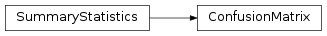# mvpa2.clfs.base.ConfusionMatrix¶class `mvpa2.clfs.base.``ConfusionMatrix`(labels=None, labels_map=None, **kwargs)

Class to contain information and display confusion matrix.

Implementation of the `SummaryStatistics` in the case of classification problem. Actual computation of confusion matrix is delayed until all data is acquired (to figure out complete set of labels). If testing data doesn’t have a complete set of labels, but you like to include all labels, provide them as a parameter to the constructor.

Confusion matrix provides a set of performance statistics (use as_string(description=True) for the description of abbreviations), as well ROC curve (http://en.wikipedia.org/wiki/ROC_curve) plotting and analysis (AUC) in the limited set of problems: binary, multiclass 1-vs-all.

Attributes

 `error` `labels` `labels_map` `matrices` Return a list of separate confusion matrix per each stored set `matrix` `percent_correct` `sets` `stats` `summaries` Return a list of separate summaries per each stored set

Methods

 `__call__`(predictions, targets[, estimates, ...]) Computes confusion matrix (counts) `add`(targets, predictions[, estimates]) Add new results to the set of known results `as_string`([short, header, summary, description]) ‘Pretty print’ the matrix `compute`() Actually compute the confusion matrix based on all the sets `get_labels_map`() `plot`([labels, numbers, origin, ...]) Provide presentation of confusion matrix in image `reset`() Cleans summary – all data/sets are wiped out `set_labels_map`(val)

Initialize ConfusionMatrix with optional list of `labels`

Parameters: labels : list Optional set of labels to include in the matrix labels_map : None or dict Dictionary from original dataset to show mapping into numerical labels targets Optional set of targets predictions Optional set of predictions

Attributes

 `error` `labels` `labels_map` `matrices` Return a list of separate confusion matrix per each stored set `matrix` `percent_correct` `sets` `stats` `summaries` Return a list of separate summaries per each stored set

Methods

 `__call__`(predictions, targets[, estimates, ...]) Computes confusion matrix (counts) `add`(targets, predictions[, estimates]) Add new results to the set of known results `as_string`([short, header, summary, description]) ‘Pretty print’ the matrix `compute`() Actually compute the confusion matrix based on all the sets `get_labels_map`() `plot`([labels, numbers, origin, ...]) Provide presentation of confusion matrix in image `reset`() Cleans summary – all data/sets are wiped out `set_labels_map`(val)
`as_string`(short=False, header=True, summary=True, description=False)

‘Pretty print’ the matrix

Parameters: short : bool if True, ignores the rest of the parameters and provides consise 1 line summary header : bool print header of the table summary : bool print summary (accuracy) description : bool print verbose description of presented statistics
`error`
`get_labels_map`()
`labels`
`labels_map`
`matrices`

Return a list of separate confusion matrix per each stored set

`matrix`
`percent_correct`
`plot`(labels=None, numbers=False, origin='upper', numbers_alpha=None, xlabels_vertical=True, numbers_kwargs=None, **kwargs)

Provide presentation of confusion matrix in image

Parameters: labels : list of int or str Optionally provided labels guarantee the order of presentation. Also value of None places empty column/row, thus provides visual groupping of labels (Thanks Ingo) numbers : bool Place values inside of confusion matrix elements numbers_alpha : None or float Controls textual output of numbers. If None – all numbers are plotted in the same intensity. If some float – it controls alpha level – higher value would give higher contrast. (good value is 2) origin : str Which left corner diagonal should start xlabels_vertical : bool Either to plot xlabels vertical (benefitial if number of labels is large) numbers_kwargs : dict Additional keyword parameters to be added to numbers (if numbers is True) **kwargs Additional arguments given to imshow (eg me cmap) (fig, im, cb) – figure, imshow, colorbar
`set_labels_map`(val)